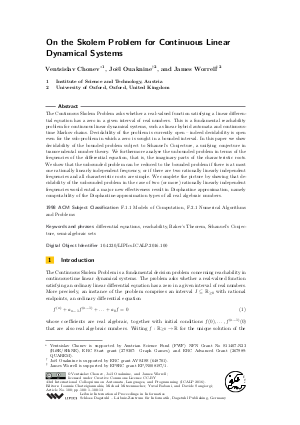Document# On the Skolem Problem for Continuous Linear Dynamical Systems

### Authors Ventsislav Chonev, Joël Ouaknine, James Worrell## File

LIPIcs.ICALP.2016.100.pdf
• Filesize: 0.49 MB
• 13 pages

## Cite As

Ventsislav Chonev, Joël Ouaknine, and James Worrell. On the Skolem Problem for Continuous Linear Dynamical Systems. In 43rd International Colloquium on Automata, Languages, and Programming (ICALP 2016). Leibniz International Proceedings in Informatics (LIPIcs), Volume 55, pp. 100:1-100:13, Schloss Dagstuhl - Leibniz-Zentrum für Informatik (2016)
https://doi.org/10.4230/LIPIcs.ICALP.2016.100

## Abstract

The Continuous Skolem Problem asks whether a real-valued function satisfying a linear differential equation has a zero in a given interval of real numbers. This is a fundamental reachability problem for continuous linear dynamical systems, such as linear hybrid automata and continuoustime Markov chains. Decidability of the problem is currently open — indeed decidability is open even for the sub-problem in which a zero is sought in a bounded interval. In this paper we show decidability of the bounded problem subject to Schanuel's Conjecture, a unifying conjecture in transcendental number theory. We furthermore analyse the unbounded problem in terms of the frequencies of the differential equation, that is, the imaginary parts of the characteristic roots. We show that the unbounded problem can be reduced to the bounded problem if there is at most one rationally linearly independent frequency, or if there are two rationally linearly independent frequencies and all characteristic roots are simple. We complete the picture by showing that decidability of the unbounded problem in the case of two (or more) rationally linearly independent frequencies would entail a major new effectiveness result in Diophantine approximation, namely computability of the Diophantine-approximation types of all real algebraic numbers.
##### Keywords
• differential equations
• reachability
• Baker’s Theorem
• Schanuel’s Conjecture
• semi-algebraic sets

## Metrics

• Access Statistics
• Total Accesses (updated on a weekly basis)
0

## References

1. Rajeev Alur. Principles of Cyber-Physical Systems. MIT Press, 2015.2. Alan Baker. Transcendental number theory. Cambridge University Press, Cambridge, 1975.3. Saugata Basu, Richard Pollack, and Marie-Françoise Roy. Algorithms in Real Algebraic Geometry (Algorithms and Computation in Mathematics). Springer-Verlag, 2006.4. Paul C. Bell, Jean-Charles Delvenne, Raphaël M. Jungers, and Vincent D. Blondel. The Continuous Skolem-Pisot Problem. Theoretical Computer Science (TCS), 411(40-42):3625-3634, 2010.5. Edward Bierstone and Pierre D. Milman. Semianalytic and subanalytic sets. Publications Mathématiques de l'Institut des Hautes Études Scientifiques, 67(1):5-42, 1988.6. Richard P. Brent. Fast multiple-precision evaluation of elementary functions. Journal of the ACM (JACM), 23(2):242-251, 1976.7. Ventsislav Chonev, Joël Ouaknine, and James Worrell. On the skolem problem for continuous linear dynamical systems. CoRR, abs/1506.00695, 2015.8. Ventsislav Chonev, Joël Ouaknine, and James Worrell. On recurrent reachability for continuous linear dynamical systems. Logic in Computer Science (LICS), 2016.9. Henri Cohen. A Course in Computational Algebraic Number Theory. Springer-Verlag, 1993.10. Paul M. Cohn. Basic Algebra: Groups, Rings and Fields. Springer, 2002.11. David A. Cox, John Little, and Donal O'Shea. Ideals, Varieties, and Algorithms: An Introduction to Computational Algebraic Geometry and Commutative Algebra. Springer, 2007.12. Vesa Halava, Tero Harju, Mika Hirvensalo, and Juhani Karhumäki. Skolem’s Problem - on the Border between Decidability and Undecidability. TUCS Technical Reports, 683, 2005.13. Godfrey H. Hardy and Edward M. Wright. An Introduction to the Theory of Numbers, volume 1. Oxford, 1999.14. Bettina Just. Integer relations among algebraic numbers. In Mathematical Foundations of Computer Science (MFCS), volume 379, pages 314-320. Springer, 1989.15. Serge Lang. Introduction to Transcendental Numbers. Reading, Mass., 1966.16. Arjen K. Lenstra. Factoring multivariate polynomials over algebraic number fields. SIAM Journal on Computing, 16(3):591-598, 1987.17. Angus Macintyre. Turing meets Schanuel. Preprint, to appear in the proceedings of Logic Colloquium, 2012.18. Angus Macintyre and Alex J. Wilkie. On the decidability of the real exponential field. In (ed. Piergiorgio Odifreddi) Kreiseliana: About and Around Georg Kreisel., 1996.19. Victor Y. Pan. Optimal and nearly optimal algorithms for approximating polynomial zeros. Computers and Mathematics with Applications, 31(12):97-138, 1996.20. Terence Tao. Structure and randomness: pages from year one of a mathematical blog. American Mathematical Society, 2008.21. Boris Zilber. Exponential sums equations and the Schanuel conjecture. Journal of the London Mathematical Society, 65:27-44, 2002.22. Boris Zilber. Pseudo-exponentiation on algebraically closed fields of characteristic zero. Annals of Pure and Applied Logic, 132(1):67-95, 2005.X

Feedback for Dagstuhl Publishing